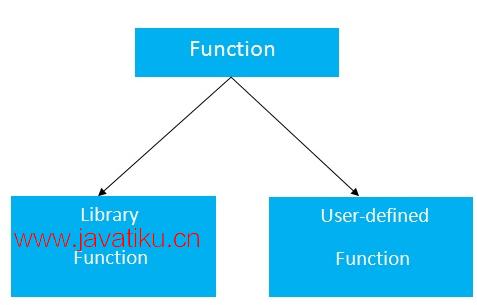# C++教程-C++数组## C++ 数组• 代码优化（减少代码量）
• 随机访问
• 易于遍历数据
• 易于操作数据
• 易于对数据进行排序等等

• 大小固定

1. 一维数组
2. 多维数组

### C++ 一维数组

``````\#include <iostream>
using namespace std;
int main()
{
int arr={10, 0, 20, 0, 30}; // 创建和初始化数组
// 遍历数组
for (int i = 0; i < 5; i++)
{
cout<<arr[i]<<"\n";
}
}``````

``````10
0
20
0
30 ``````

### C++ 数组示例：使用 foreach 循环遍历

``````\#include <iostream>
using namespace std;
int main()
{
int arr={10, 0, 20, 0, 30}; // 创建和初始化数组
// 遍历数组
for (int i: arr)
{
cout<<i<<"\n";
}
}``````

``````10
20
30
40
50 ``````

### C++ 数组中的空成员

``// 在数组中只存储 3 个元素 int x = {19, 10, 8}; ``

### 在使用数组时需要记住的重要事项

1. 数组的索引从 0 开始。这意味着第一个保存在索引 0 处的项是 x。
2. 大小为 n 的数组的最后一个元素保存在索引 (n-1) 处。此示例的最后一个元素是 x。
3. 数组的元素具有连续的地址。考虑 x 的地址为 2120 的情况。

### 如何将元素插入数组中？

``````int mark = {19, 10, 8, 17, 9};
// 将第4个元素改为9
mark = 9;
// 从用户输入获取值
// 存储在第3个位置上
cin >> mark;
// 从用户输入获取值
// 插入在第i个位置上
cin >> mark[i-1];

// 打印数组的第一个元素
cout << mark;
// 打印数组的第i个元素
cout << mark[i-1];``````

### 如何显示数组元素的和与平均值？

``````#include <iostream>
using namespace std;

int main() {
// 不指定大小来初始化数组
double numbers[] = {7, 5, 6, 12, 35, 27};
double sum = 0;
double count = 0;
double average;

cout << "The numbers are: ";
// 打印数组元素
// 使用范围-based for 循环
for (const double &n : numbers) {
cout << n << "  ";
// 计算和
sum += n;
// 计算数组元素个数
++count;
}

// 打印和
cout << "\nTheir Sum = " << sum << endl;
// 计算平均值
average = sum / count;
cout << "Their Average = " << average << endl;

return 0;
}``````

``````The numbers are: 7 5 6 12 35 27
Their Sum = 92
Their Average = 15.3333``````

### 如何显示数组元素？

``````#include <iostream>
using namespace std;

int main() {
int numbers = {7, 5, 6, 12, 35};
cout << "The numbers are: ";
// 打印数组元素
// 使用范围-based for 循环
for (const int &n : numbers) {
cout << n << "  ";
}

cout << "\nThe numbers are: ";
// 打印数组元素
// 使用传统的 for 循环
for (int i = 0; i < 5; ++i) {
cout << numbers[i] << "  ";
}

return 0;
}``````

``````The numbers are: 7 5 6 12 35
The numbers are: 7 5 6 12 35``````